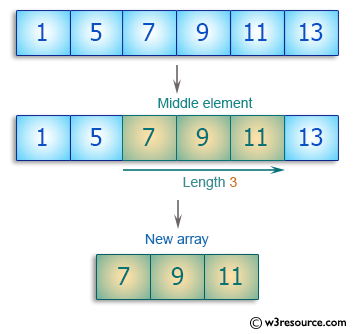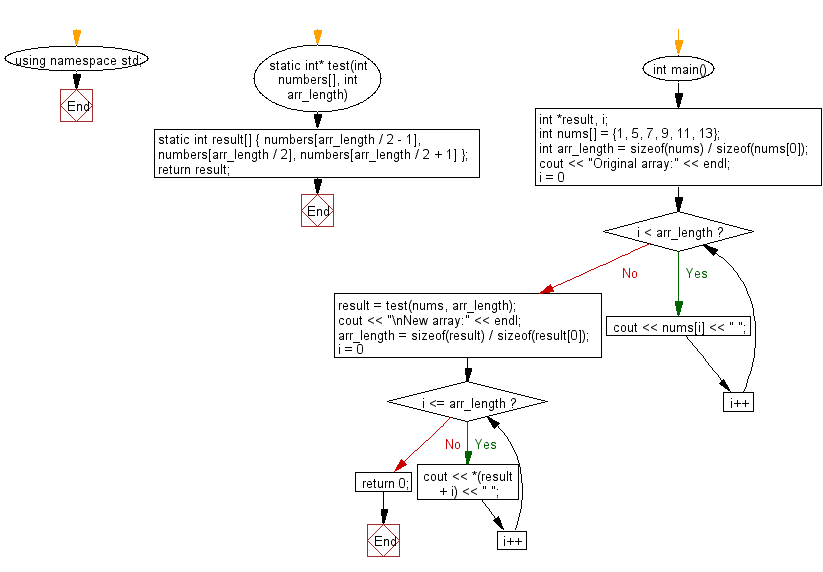﻿ C++ : New array of length 3 from the elements from an array# C++ Exercises: Create a new array length 3 from a given array the elements from the middle of the array

## C++ Basic Algorithm: Exercise-96 with Solution

Write a C++ program to create a new array length 3 from a given array (length atleast 3) using the elements from the middle of the array.

Sample Solution:

C++ Code :

``````#include <iostream>
using namespace std;

static int* test(int numbers[], int arr_length)
{
static int result[] { numbers[arr_length / 2 - 1], numbers[arr_length / 2], numbers[arr_length / 2 + 1] };
return result;
}

int main()
{
int *result, i;
int nums[] = {1, 5, 7, 9, 11, 13};
int arr_length = sizeof(nums) / sizeof(nums);

cout << "Original array:" << endl;

for ( i = 0; i < arr_length; i++ )
cout << nums[i] << " ";

result = test(nums, arr_length);
cout << "\nNew array:" << endl;
arr_length = sizeof(result) / sizeof(result);
for ( i = 0; i <= arr_length; i++ ) {
cout << *(result + i) << " ";
}

return 0;
}
``````

Sample Output:

```Original array:
1 5 7 9 11 13
New array:
7 9 11
```

Pictorial Presentation:Flowchart:C++ Code Editor:

Contribute your code and comments through Disqus.

What is the difficulty level of this exercise?

﻿